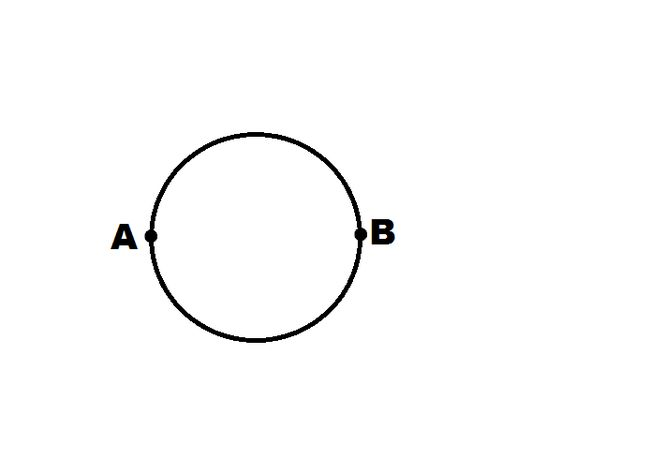# Circular resistance?The figure above shows a wire of resistance 100 $\Omega$, which is bent to form a complete circle. If $A$ and $B$ are the two diametrically opposite points, find the resistance between them in $\Omega$.

×